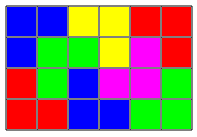CSES - Filling Trominos
• Time limit: 1.00 s
• Memory limit: 512 MB

Your task is to fill an n \times m grid using L-trominos (three squares that have an L-shape). For example, here is one way to fill a 4 \times 6 grid:# Input

The first input line has an integer t: the number of tests.

After that, there are t lines that describe the tests. Each line has two integers n and m.

# Output

For each test, print YES if there is a solution, and NO otherwise.

If there is a solution, also print n lines that each contain m letters between A–Z. Adjacent squares must have the same letter exactly when they belong to the same tromino. You can print any valid solution.

# Constraints

• 1 \le t \le 100
• 1 \le n,m \le 100

# Example

Input:

2
4 6
4 7


Output:

YES